This is an import to GitHub of the Jan. 9, 2016, version of http://finitegeometry.org/sc/.

 Finite Geometry Notes   | Home | Site Map | Author |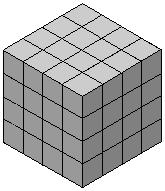### Finite Geometry of the Square and Cubeby Steven H. Cullinane

Geometry of the 4x4x4 Cube

(the mathematical structure,
not the mechanical puzzle)
and related simpler structures.

For applications of this sort
of geometry to physics, see
Quantum Information Theory.

For some of the simpler substructures of the 4x4x4 cube, see
Geometry of the 2x2 Square,
Geometry of the 2x2x2 Cube, and
Geometry of the 4x4 Square.
These traditional and supposedly well-known structures are, surprisingly, closely related to small finite geometries.

These finite geometries underlie some remarkable symmetries of graphic designs.  For instance, there is a group G of 322,560 natural transformations which, acting on the four-diamond figure D below, always yields a symmetric image.  This group turns out to also be the automorphism group of the 16-point four-dimensional finite affine space.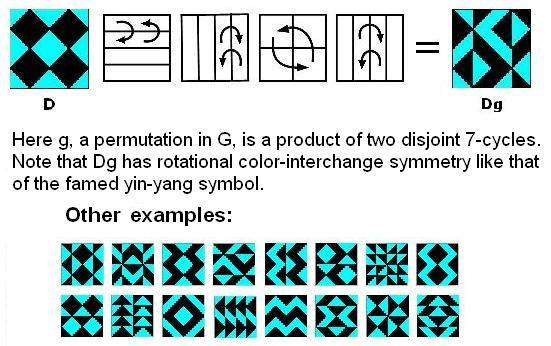Such designs are formed by assembling two-colored square tiles or two-colored cubical blocks into larger squares or cubes, when the number of tiles or blocks in the larger arrays is a power of two.

The structure underlying such graphic symmetries is that of finite projective geometry: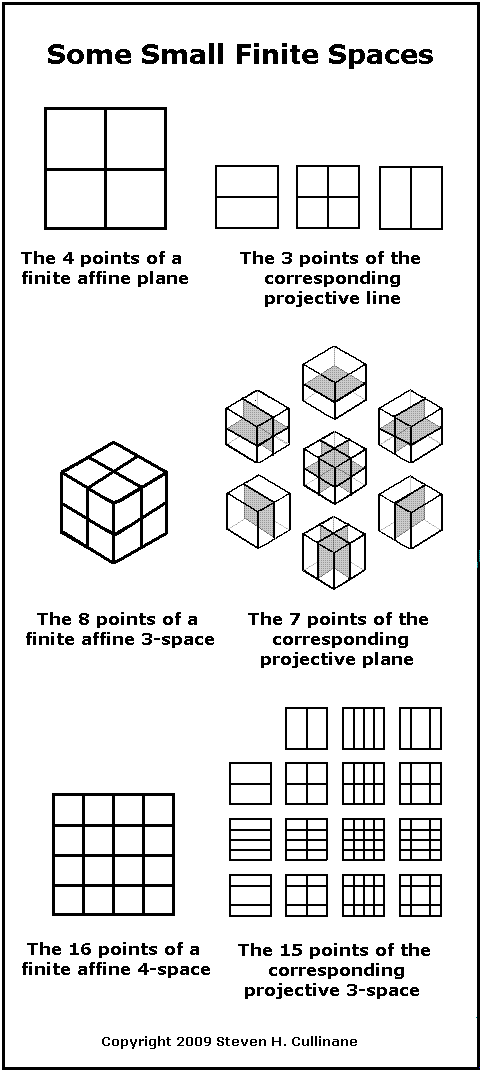Similar structural diagrams can be made for the 64 and 63 points of, respectively, finite affine and projective spaces of 6 and 5 dimensions over the 2-element field, and the graphic symmetries that result generalize the results in fewer dimensions.

For a more detailed example of how affine and projective points are related in such models, click on the image below.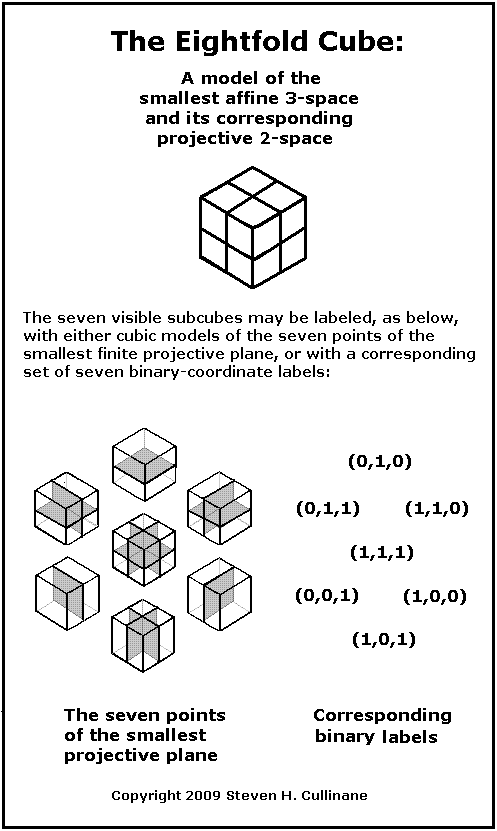An earlier presentation
of the above seven partitions
of the eightfold cube: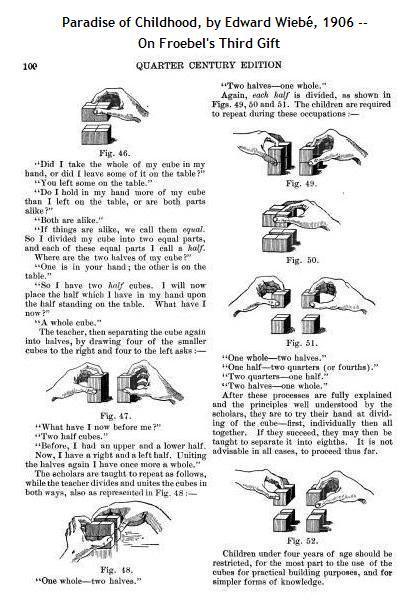When the number of tiles or blocks in a square or cubical array is a power of an odd prime, symmetry of a different sort results.  In the power-of-two case, despite the designs' overall symmetry,  the natural permutations interchanging tiles or blocks are generally asymmetric.  In the odd-prime case, there is no natural way to form symmetric graphic designs, but, on the other hand, the natural permutations of tiles or blocks are themselves always symmetric.

For the some of the simplest examples of the odd-prime case, see

Geometry of the 3x3 Square
and
Geometry of the 3x3x3 Cube.

For a more detailed look at these topics, see

Notes on Finite Geometry (the detailed site map for this website).

 Some Context Towards a Philosophy of Real Mathematics, by David Corfield, Cambridge U. Press, 2003, p. 206: “Now, it is no easy business defining what one means by the term conceptual…. I think we can say that the conceptual is usually expressible in terms of broad principles. A nice example of this comes in the form of harmonic analysis, which is based on the idea, whose scope has been shown by George Mackey (1992) to be immense, that many kinds of entity become easier to handle by decomposing them into components belonging to spaces invariant under specified symmetries.” For a simpler example of this idea, see the entities in The Diamond Theorem, the decomposition in A Four-Color Theorem, and the space in Geometry of the 4×4 Square. The decomposition differs from that of harmonic analysis, although the subspaces involved in the diamond theorem are isomorphic to Walsh functions– well-known as discrete analogues of the trigonometric functions of traditional harmonic analysis.

f harmonic analysis, although the subspaces involved in the diamond theorem are isomorphic to Walsh functions– well-known as discrete analogues of the trigonometric functions of traditional harmonic analysis.